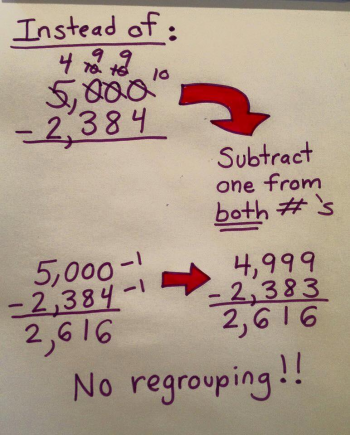Games
Problems
Go Pro!

Sherry from Los Angeles asks, "I read that the method for subtracting without regrouping works all the time. But what about a problem like 6563 - 1998. You can take 1 away from each but still need to regroup. I’m a teacher and got so excited when I saw this, but it doesn’t always work??"Hi Sherry, you're probably referencing this meme here. This meme shows subtracting a quantity from a number that has all zeroes after the first digit. Does this always work? Yes it does. Because if all the digits are zeroes, subtracting one from the number turns all the zeroes into nines, which means you don't ever have to regroup/borrow to finish the problem.

But that's only if those digits are all zeroes to begin with. What are the real world situations where this is likely to happen? If you're making change at a store. If you've paid with a 50 dollar bill, and the cost of the item is \$24.32, your change would be \$50.00 - 24.32. You can simplify this problem by rewriting it as \$49.99 - 24.31 = \$25.68.

Your question is, essentially, what if the first number doesn't end with all zeroes after the first digit?

And the answer is, it still works, but is not as likely to be practical. Here's an example: 1002 - 865. What we want to do is change the first quantity so it ends with nines. To do that, we subtract three instead of one. Which means, of course, that we have to subtract three from the second quantity as well: 999 - 862 = 137.

So now we get to your example: 6563 - 1998. We ask ourselves, what would we need to subtract from the first quantity to make it end in nines? Answer: 564. But that means we have to subtract 564 from the second quantity as well. In this particular case, we can do that subtraction without regrouping: 1998 - 564 = 1434. So 6563 - 1998 = 5999 - 1434 = 4565. But if regrouping had been required at the second step, we would have had to do our process again, and we'd end up with a cascading series of subtractions which would have been far more ugly than just regrouping in the first place.

As a general rule of thumb, even though it does (theoretically) work for problems like the one you suggested, in practice, it's very cumbersome, and I wouldn't recommend doing it that way.Like us on Facebook to get updates about new resources